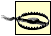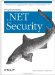# 13.4 Programming Keyed Hashing Algorithms

The .NET Framework has a much more direct model for representing keyed hashing algorithms. There are no abstract classes for different algorithms, meaning that there can be only one implementation of each keyed algorithm. Figure 13-7 shows the class hierarchy for keyed hashing algorithms.

##### Figure 13-7. The .NET Framework class hierarchy for keyed hashing algorithms.#### 13.4.1 The KeyedHashAlgorithm Class

The KeyedHashAlgorithm class extends HashAlgorithm, adding a new property called Key, which is used to get and set the secret key as a byte array. Aside from this addition, the KeyedHashAlgorithm class provides the same abstraction benefits as using HashAlgorithm for creating normal hash codes.

#### 13.4.2 Instantiating the Algorithm

The process of creating instances of keyed algorithms is much the same as for normal hashing algorithms. You can create algorithms directly using the implementation class name, or indirectly using the Create method of the KeyedHashingAlgorithm class.

When using the direct approach, you can supply the secret key as an argument to the class constructor. In the following fragment, use the System.Text.Encoding class to convert a string into a byte array and to initialize the keyed hashing algorithm:

`# C# // define the key as a string string x_key_string = "This is my secret key"; // convert the string to a byte array byte[] x_key_bytes       = System.Text.Encoding.Default.GetBytes(x_key_string); // create the keyed hashing algorithm, using the  // byte array as the constructor argument KeyedHashAlgorithm x_hash_alg = new HMACSHA1(x_key_bytes); # Visual Basic .NET ' define the key as a string Dim x_key_string As String = " This is my secret key " ' convert the string to a byte array Dim x_key_bytes As Byte(  ) _     = System.Text.Encoding.Default.GetBytes(x_key_string) ' create the keyed hashing algorithm, using the  ' byte array as the constructor argument Dim x_hash_alg As KeyedHashAlgorithm = New HMACSHA1(x_key_bytes)`

These statements create an instance of the HMAC-SHA-1 implementation class, using the key "This is my secret key." When using the Create method, the key value is set separately, using the Key property. The following fragment demonstrates how to do this:

`# C# // create the keyed hashing algorithm, using the  // byte array as the constructor argument KeyedHashAlgorithm x_hash_alg = KeyedHashAlgorithm.Create("HMACSHA1"); // define the key as a string string x_key_string = " This is my secret key "; // convert the string to a byte array byte[] x_key_bytes       = System.Text.Encoding.Default.GetBytes(x_key_string); // set the keyed hash algorithm key x_hash_alg.Key = x_key_bytes; # Visual Basic .NET ' create the keyed hashing algorithm, using the  ' byte array as the constructor argument Dim x_hash_alg As KeyedHashAlgorithm = KeyedHashAlgorithm.Create("HMACSHA1") ' define the key as a string Dim x_key_string As String = " This is my secret key " ' convert the string to a byte array Dim x_key_bytes As Byte(  ) _     = System.Text.Encoding.Default.GetBytes(x_key_string) ' set the keyed hash algorithm key x_hash_alg.Key = x_key_bytes`

The Create method creates an algorithm class based on the value of the string argument. Table 13-4 lists the mapping between string values and implementation classes for keyed hashing algorithms.If you do not supply a string as an argument to the Create method, the System.Security.Cryptography.HMACSHA1 algorithm will be used as a default. If you use a string that is not one of the values listed in Table 13-4, then a System.NullReferenceException will be thrown.

##### Table 13-4. Mapping string values to keyed algorithm classes

String value

Algorithm implementation class

HMACSHA1

HMACSHA1

System.Security.Cryptography.HMACSHA1

HMACSHA1

MACTripleDES

MACTripleDES

System.Security.Cryptography.MACTripleDES

MACTripleDES

#### 13.4.3 Hashing Data and Validating Hash Codes

Because the KeyedHashAlgorithm class derives from HashAlgorithm, the processes for creating and validating keyed hash codes is the same as for normal hash codes, with the exception that the value of the key should be set either as a constructor argument or as using the Key property. For reference, Example 13-2 demonstrates how to create a keyed hash code from a byte array of data:You must set the secret key value before creating the hash code; otherwise, the KeyedHashAlgorithm will create a random key. See Chapter 17 for more information about creating and managing keys.

##### Example 13-2. Creating a keyed hash code from a byte array of data
`# C# using System; using System.Text; using System.Security.Cryptography; class ByteArrayHash {     static void Main(string[] args) {                  // define the string that we will         // create a hash code for         String x_str = "Programming .NET Security";         // create a byte array from the string         byte[] x_message_data = Encoding.Default.GetBytes(x_str);         // define the secret key as a string         string x_key_string = "This is my secret key";         // create a byte array containing the key         byte[] x_key_bytes = Encoding.Default.GetBytes(x_key_string);         // create an instance of the HMAC-SHA-1 keyed algorithm         KeyedHashAlgorithm x_hash_alg = KeyedHashAlgorithm.Create("HMACSHA1");         // set the secret key for the hashing algorithm         x_hash_alg.Key = x_key_bytes;         // obtain the hash code from the HashAlgorithm by          // using the ComputeHash method         byte[] x_hash_code = x_hash_alg.ComputeHash(x_message_data);         // print out the hash code to the console         foreach (byte x_byte in x_hash_code) {             Console.Write("{0:X2} ", x_byte);         }     } } # Visual Basic .NET Imports System Imports System.Text Imports System.Security.Cryptography Module Module1     Sub Main(  )         ' define the string that we will         ' create a hash code for         Dim x_str As String = "Programming .NET Security"         ' create a byte array from the string         Dim x_message_data(  ) As Byte = Encoding.Default.GetBytes(x_str)         ' define the secret key as a string         Dim x_key_string As String = "This is my secret key"         ' create a byte array containing the key         Dim x_key_bytes(  ) As Byte = Encoding.Default.GetBytes(x_key_string)         ' create an instance of the HMAC-SHA-1 keyed algorithm         Dim x_hash_alg As KeyedHashAlgorithm = _             KeyedHashAlgorithm.Create("HMACSHA1")         ' set the secret key for the hashing algorithm         x_hash_alg.Key = x_key_bytes         ' obtain the hash code from the HashAlgorithm by          ' using the ComputeHash method         Dim x_hash_code(  ) As Byte = x_hash_alg.ComputeHash(x_message_data)         ' print out the hash code to the console         Dim x_byte As Byte         For Each x_byte In x_hash_code             Console.Write("{0:X2} ", x_byte)         Next     End Sub End Module`Programming .Net Security
ISBN: 0596004427
EAN: 2147483647
Year: 2005
Pages: 346

Similar book on Amazon

flylib.com © 2008-2017.
If you may any questions please contact us: flylib@qtcs.net